Calculation groups are a new feature in DAX, inspired from a similar feature available in MDX known as calculated members. Calculation groups are easy to use; however, designing a model with calculation groups correctly can be challenging when you create multiple calculation groups or when you use calculation items in measures.

Before we provide a description of calculation groups, it is useful to spend some time analyzing the business requirement that led to the introduction of this feature. An example involving time-related calculations fits perfectly well.

Our sample model contains measures to compute the sales amount, the total cost, the margin, and the total quantity sold by using the following DAX code:

```Sales Amount   := SUMX ( Sales, Sales[Quantity] * Sales[Net Price] )
Total Cost     := SUMX ( Sales, Sales[Quantity] * Sales[Unit Cost] )
Margin         := [Sales Amount] - [Total Cost]
Sales Quantity := SUM ( Sales[Quantity] )
```

All four measures are useful, and they provide different insights into the business. Moreover, all four measures are good candidates for time intelligence calculations. A year-to-date over sales quantity can be as interesting as a year-to-date over sales amount and over margin. The same consideration is true for many other time intelligence calculations: same period last year, growth in percentage against the previous year, and many others.

Nevertheless, if one wants to build all the different time intelligence calculations for all the measures, the number of measures in the data model may grow very quickly. In the real world, managing a data model with hundreds of measures is intimidating for both users and developers. Finally, consider that all the different measures for time intelligence calculations are simple variations of a common pattern. For example, the year-to-date versions of the previous list of four measures would look like the following:

```YTD Sales Amount :=
CALCULATE (
[Sales Amount],
DATESYTD ( 'Date'[Date] )
)

YTD Total Cost :=
CALCULATE (
[Total Cost],
DATESYTD ( 'Date'[Date] )
)

YTD Margin :=
CALCULATE (
[Margin],
DATESYTD ( 'Date'[Date] )
)

YTD Sales Quantity :=
CALCULATE (
[Sales Quantity],
DATESYTD ( 'Date'[Date] )
)
```

All the previous measures only differ in their base measure; they all apply the same DATESYTD filter context to different base measures. It would be great if a developer were given the opportunity to define a more generic calculation, using a placeholder for the measure:

```YTD <Measure> :=
CALCULATE (
<Measure>,
DATESYTD ( 'Date'[Date] )
)
```

The previous code is not a valid DAX syntax, but it provides a very good description of what calculation items are. You can read the previous code as: When you need to apply the YTD calculation to a measure, call the measure after applying DATESYTD to the Date[Date] column. This is what a calculation item is: A calculation item is a DAX expression containing a special placeholder. The placeholder is replaced with a measure by the engine just before evaluating the result. In other words, a calculation item is a variation of an expression that can be applied to any measure.

Moreover, you will likely find yourself needing several time intelligence calculations. Indeed, year-to-date, quarter-to-date, and same period last year are all calculations that somehow belong to the same group of calculations. Therefore, DAX offers calculation items and calculation groups. A calculation group is a set of calculation items that are conveniently grouped together because they are variations on the same topic.

Let us continue with DAX pseudo-code:

```CALCULATION GROUP "Time Intelligence"
CALCULATION ITEM CY := <Measure>
CALCULATION ITEM PY := CALCULATE ( <Measure>, SAMPEPERIODLASTYEAR ( 'Date'[Date] ) )
CALCULATION ITEM QTD := CALCULATE ( <Measure>, DATESQTD ( 'Date'[Date] ) )
CALCULATION ITEM YTD := CALCULATE ( <Measure>, DATESYTD ( 'Date'[Date] ) )
```

As you can see, we grouped four time-related calculations in a group named Time Intelligence. In only four lines, the code defines dozens of different measures because the calculation items apply their variation to any measure in the model. Thus, as soon as a developer creates a new measure, the CY, PY, QTD, and YTD variations will be available at no cost.

There are still several details missing in our understanding of calculation groups, but only one is required to start taking advantage of them and to define the first calculation group: How does the user choose one variation? As we said, a calculation item is not a measure; it is a variation of a measure. Therefore, a user needs a way to put in a report a specific measure with one or more variations of the measure itself. Because users have the habit of selecting columns from tables, calculation groups are implemented as if they were columns in tables, whereas calculation items are like values of the given columns. This way, the user can use the calculation group in the columns of a matrix to display different variations of a measure in the report. For example, the calculation items previously described are applied to the columns of a matrix, showing different variations of the Sales Amount measure.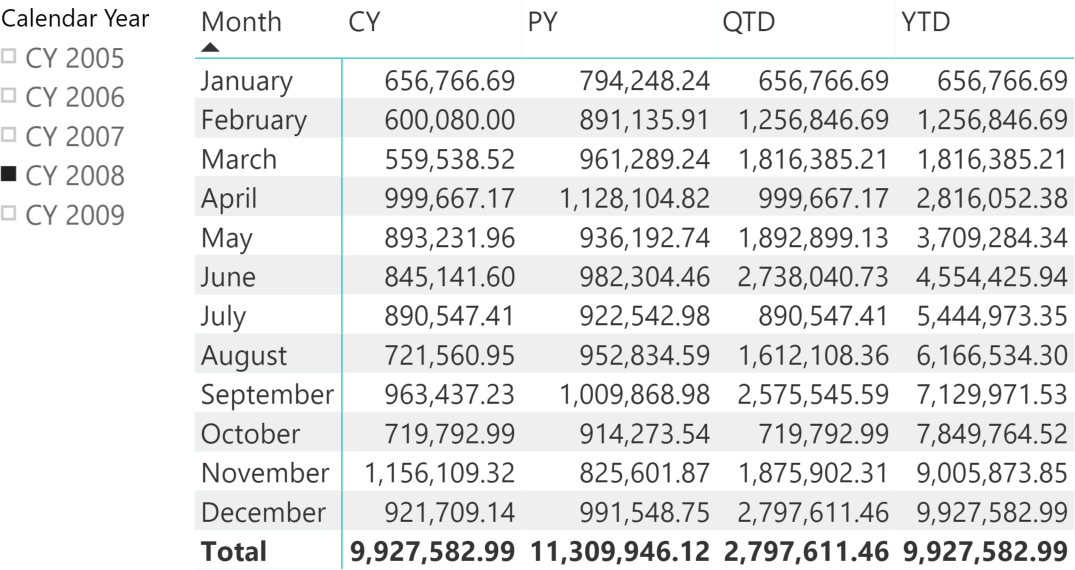## Creating calculation groups using Tabular Editor

Tabular Editor is the first tool that enables developers creating calculation groups. Because calculation groups need the compatibility version 1470 of the Tabular model, as of June 2019 they only are available in Analysis Services 2019 and Azure Analysis Services.

In Tabular Editor, the Model / New Calculation Group menu item creates a new calculation group, which appears as a table in the model with a special icon. In the following figure the calculation group has been renamed Time Intelligence.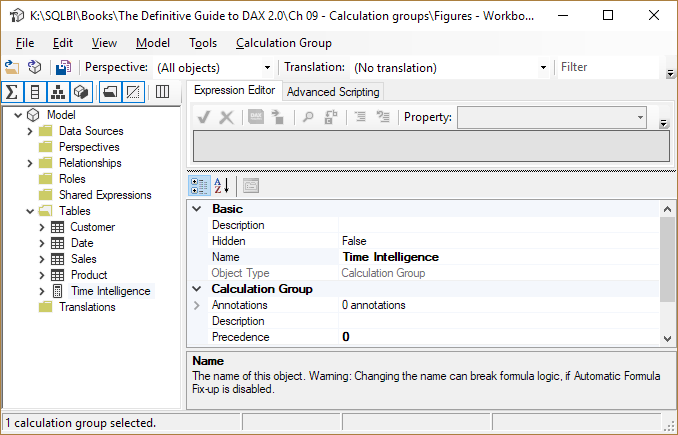A calculation group is a special table with a single column, named Attribute by default in Tabular Editor. In our sample model we renamed this column Time calc; then we added three items (YTD, QTD, and SPLY for same period last year) by using the New Calculation Item context menu item available by right-clicking on the Time calc column. Each calculation item has a DAX expression.The SELECTEDMEASURE function is the DAX implementation of the <Measure> placeholder we used in the previous DAX pseudo-code. The DAX code for each calculation item is described in the following code. The comment preceding each DAX expression identifies the corresponding calculation item:

```--
-- Calculation Item: YTD
--
CALCULATE (
SELECTEDMEASURE (),
DATESYTD ( 'Date'[Date] )
)

--
-- Calculation Item: QTD
--
CALCULATE (
SELECTEDMEASURE (),
DATESQTD ( 'Date'[Date] )
)

--
-- Calculation Item: SPLY
--
CALCULATE (
SELECTEDMEASURE (),
SAMEPERIODLASTYEAR ( 'Date'[Date] )
)
```

With this definition, the user sees a new table named Time Intelligence, with a column named Time calc containing three values: YTD, QTD, and SPLY. The user can create a slicer on that column or use it on the rows and columns of visuals, as if it were a real column in the model. For example, when the user selects YTD, the engine applies the YTD calculation item to whatever measure is in the report. The next figure shows a matrix containing the Sales Amount measure. Because the slicer selects the YTD variation of the measure, the numbers shown are year-to-date values.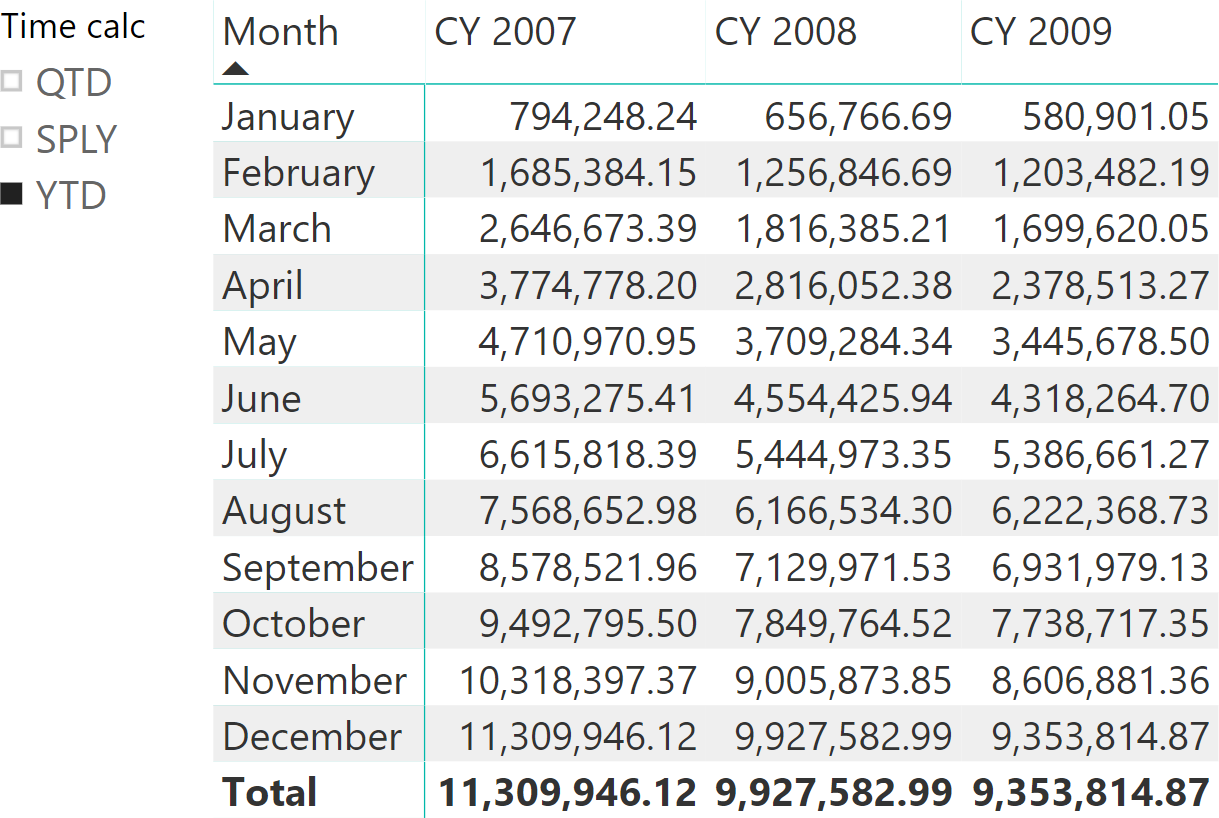If on the same report the user selects SPLY, the result will be very different.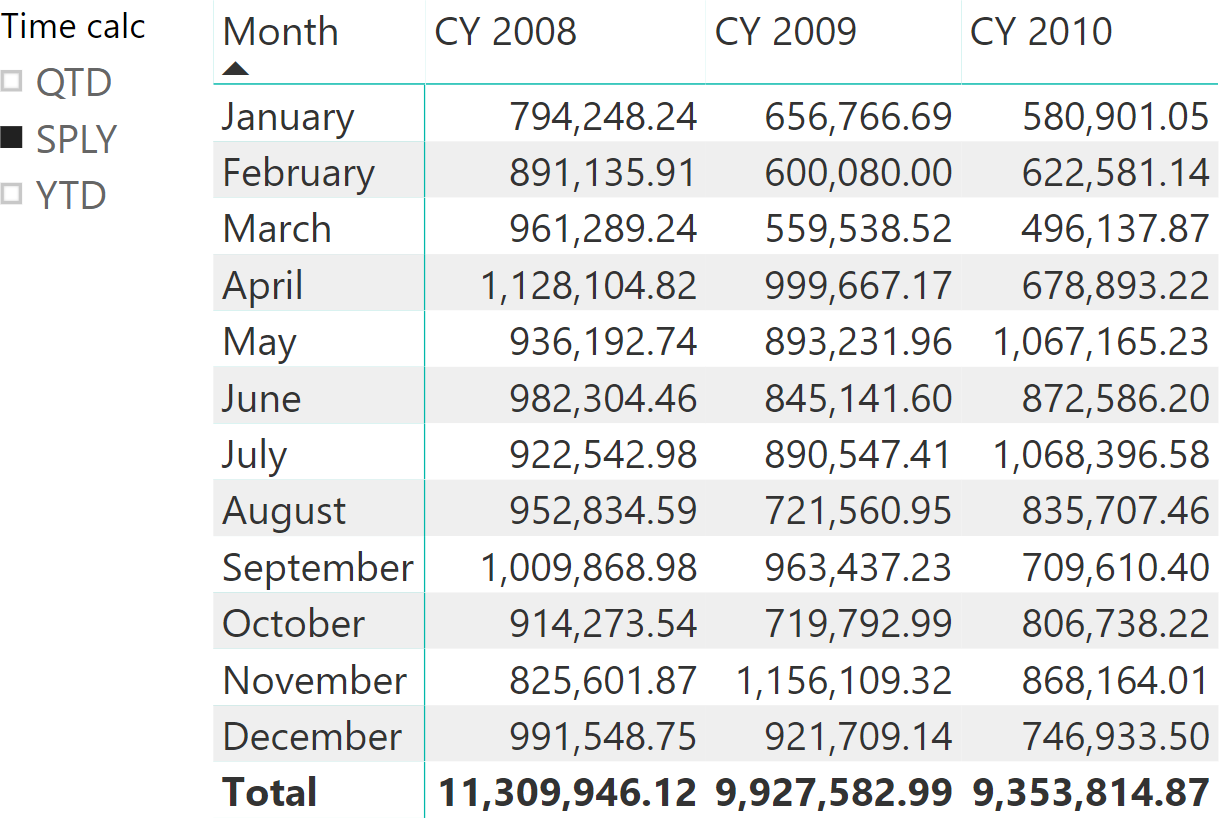If the user does not select one value or if the user selects multiple values together, then the engine does not apply any variation to the original measure.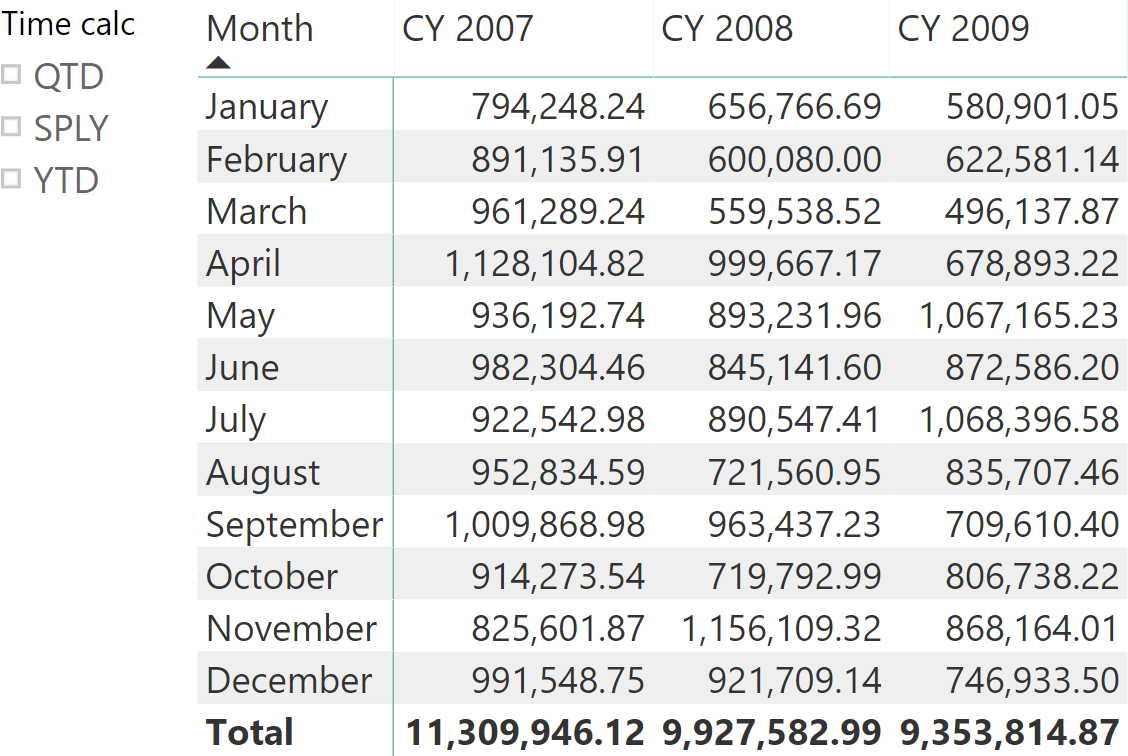Calculation groups can go further than that. At the beginning of this article we introduced four different measures: Sales Amount, Total Cost, Margin, and Sales Quantity. It would be extremely nice if the user could use a slicer in order to select the metric to show and not only the time intelligence calculation to apply. We would like to present a generic report that slices any of the four metrics by month and year, letting the user choose the desired metric. In other words, we want to obtain the report below.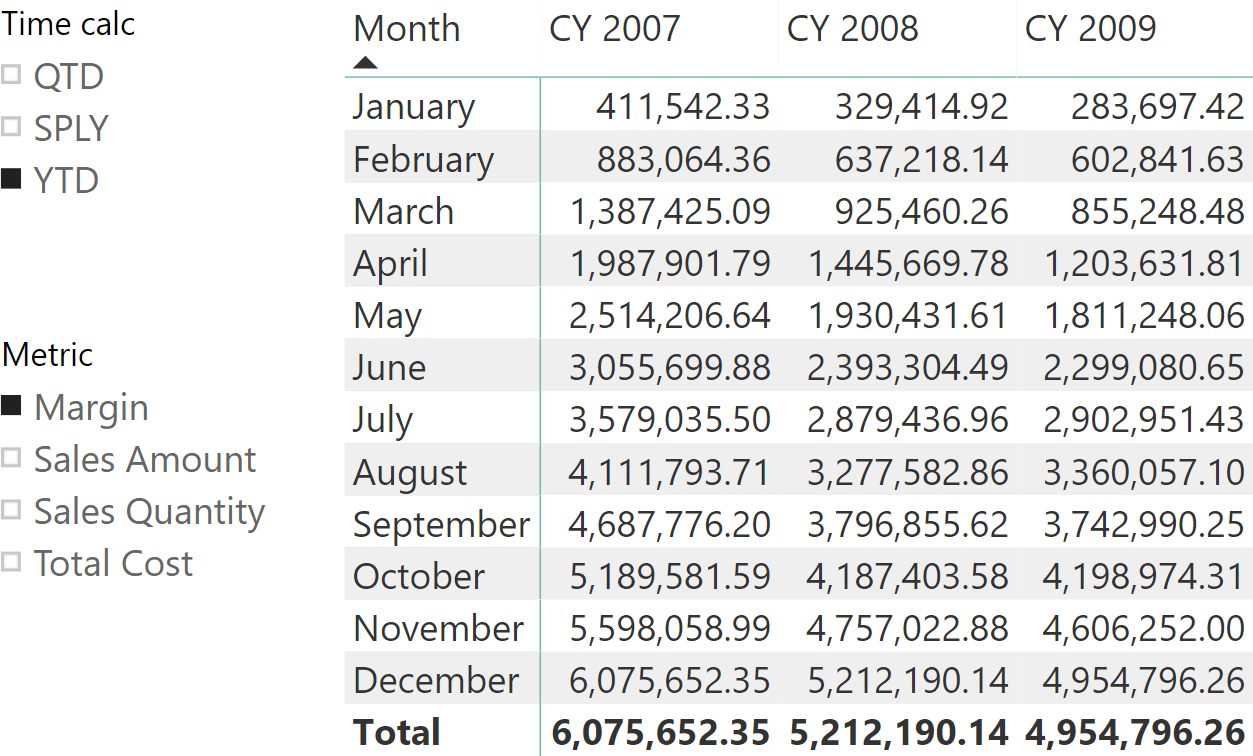In the example shown, the user is browsing the margin amount using a year-to-date variation. Nevertheless, the user can choose any combination of the slicers linked to the two calculation groups, Metric and Time calc.

In order to obtain this report, we created an additional calculation group named Metric, which includes the Sales Amount, Total Cost, Margin, and Sales Quantity calculation items. The expression for each calculation item just evaluates the corresponding measure.When there are multiple calculation groups in the same data model, it is important to define in which order they should be applied by the DAX engine. The Precedence property of the calculation groups defines the order of application: the first calculation group applied is the one with the larger value. In order to obtain the desired result, we increased the Precedence property of the Time Intelligence calculation group to 10.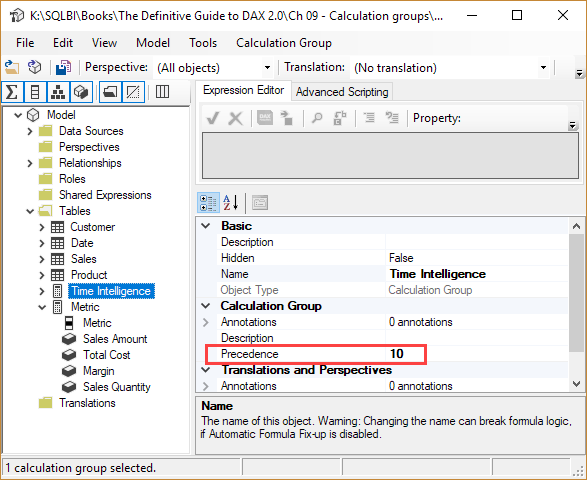As a consequence, the engine applies the Time Intelligence calculation group before the Metric calculation group, which keeps the Precedence property at the default value of zero. The following DAX code includes the definition of each calculation item in the Metric calculation group:

```--
-- Calculation Item: Margin
--
[Margin]

--
-- Calculation Item: Sales Amount
--
[Sales Amount]

--
-- Calculation Item: Sales Quantity
--
[Sales Quantity]

--
-- Calculation Item: Total Cost
--
[Total Cost]
```

These calculation items are not modifiers of the original measure. Instead, they completely replace the original measure with a new one. To obtain this behavior, we omitted a reference to SELECTEDMEASURE in the expression. SELECTEDMEASURE is used very often in calculation items, but it is not mandatory.

## Including and excluding measures from calculation items

There are scenarios where a calculation item implements a variation that does not make sense on all the measures. By default, a calculation item applies its effects on all the measures. Nevertheless, the developer might want to restrict which measures are affected by a calculation item.

One can write conditions in DAX that analyze the current measure evaluated in the model by using either ISSELECTEDMEASURE or SELECTEDMEASURENAME . The ISSELECTEDMEASURE function returns TRUE if the measure evaluated by SELECTEDMEASURE is included in the list of measures specified in the arguments. For example, the following code applies the calculation item to any measure, except to the Margin % measure:

```IF (
NOT ISSELECTEDMEASURE ( [Margin %] ),
DIVIDE (
SELECTEDMEASURE (),
COUNTROWS ( 'Date' )
)
)
```

Another function that can be used to analyze the selected measure in a calculation item expression is SELECTEDMEASURENAME, which returns a string instead of a Boolean value. For example, the previous code can be written also this way:

```IF (
NOT ( SELECTEDMEASURENAME () = "Margin %" ),
DIVIDE (
SELECTEDMEASURE (),
COUNTROWS ( 'Date' )
)
)
```

ISSELECTEDMEASURE is preferable over SELECTEDMEASURENAME for several reasons:

• If the measure name is misspelled using a comparison with SELECTEDMEASURENAME, the DAX code simply return FALSE without raising an error.
• If the measure name is misspelled using ISSELECTEDMEASURE, the expression fails with the error Invalid input arguments for ISSELECTEDMEASURE .
• If a measure is renamed in the model, all the expressions using ISSELECTEDMEASURE are automatically renamed in the model editor (formula fixup), whereas the strings compared to SELECTEDMEASURENAME must be updated manually.

The SELECTEDMEASURENAME function should be considered when the business logic of a calculation item must apply a transformation based on an external configuration. For example, the function might be useful when there is a table with a list of measures that should enable a behavior in a calculation item so that the model has an external configuration that can be modified without requiring an update of the DAX code.

## Conclusions

Calculation groups are a new and exciting feature of DAX. In this first article we just introduced calculation groups. Following articles describe in more details the properties available, several examples of possible usages and the best practice to write reliable code.